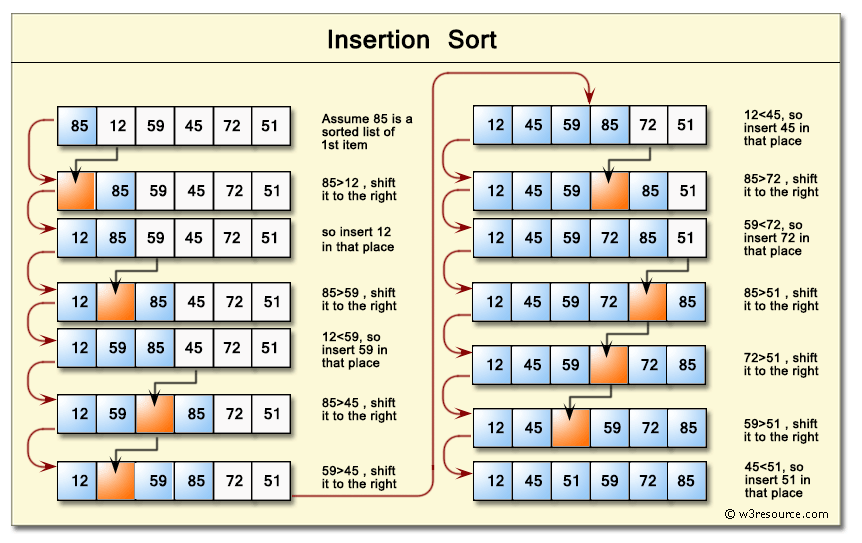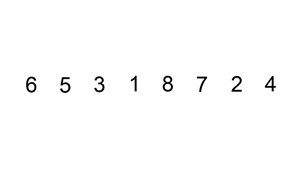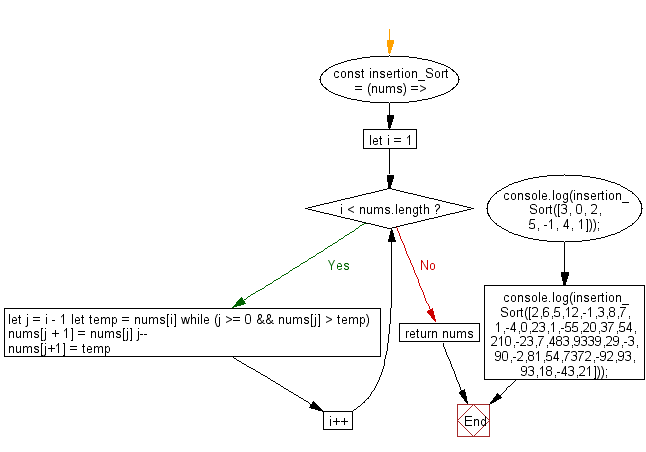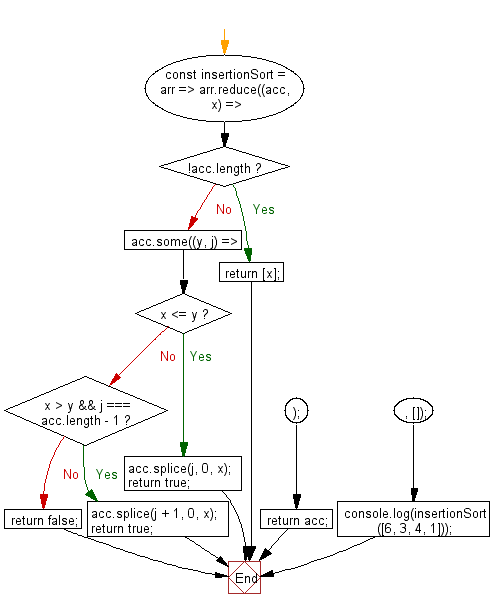# JavaScript Sorting Algorithm: Sorts an array of numbers, using the insertion sort algorithm

## JavaScript Sorting Algorithm: Exercise-4 with Solution

Write a JavaScript program to sort a list of elements using Insertion sort.

Insertion sort is a simple sorting algorithm that builds the final sorted array (or list) one item at a time. It is much less efficient on large lists than more advanced algorithms such as quicksort, heapsort, or merge sort.

Pictorial Presentation: Insertion SortA graphical example of insertion sort:Animation Credits: Swfung8

Sample Solution-1:

JavaScript Code:

``````const insertion_Sort = (nums) => {
for (let i = 1; i < nums.length; i++) {
let j = i - 1
let temp = nums[i]
while (j >= 0 && nums[j] > temp) {
nums[j + 1] = nums[j]
j--
}
nums[j+1] = temp
}
return nums
}
console.log(insertion_Sort([3, 0, 2, 5, -1, 4, 1]));
console.log(insertion_Sort([2,6,5,12,-1,3,8,7,1,-4,0,23,1,-55,20,37,54,210,-23,7,483,9339,29,-3,90,-2,81,54,7372,-92,93,93,18,-43,21]));
```
```

Sample Output:

```[-1,0,1,2,3,4,5]
[-92,-55,-43,-23,-4,-3,-2,-1,0,1,1,2,3,5,6,7,7,8,12,18,20,21,23,29,37,54,54,81,90,93,93,210,483,7372,9339]
```

Flowchart:Sample Solution-2:

• Use Array.prototype.reduce() to iterate over all the elements in the given array.
• If the length of the accumulator is 0, add the current element to it.
• Use Array.prototype.some() to iterate over the results in the accumulator until the correct position is found.
• Use Array.prototype.splice() to insert the current element into the accumulator.

JavaScript Code:

``````const insertionSort = arr =>
arr.reduce((acc, x) => {
if (!acc.length) return [x];
acc.some((y, j) => {
if (x <= y) {
acc.splice(j, 0, x);
return true;
}
if (x > y && j === acc.length - 1) {
acc.splice(j + 1, 0, x);
return true;
}
return false;
});
return acc;
}, []);

console.log(insertionSort([6, 3, 4, 1]));
```
```

Sample Output:

```[1,3,4,6]
```

Flowchart:Live Demo:

See the Pen searching-and-sorting-algorithm-exercise-4 by w3resource (@w3resource) on CodePen.

Improve this sample solution and post your code through Disqus

What is the difficulty level of this exercise?

Test your Programming skills with w3resource's quiz.

﻿